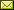## A Graphical Calculus for Lagrangian Relations

 Cole Comfort (University of Oxford) Aleks Kissinger (University of Oxford)

 Symplectic vector spaces are the phase spaces of linear mechanical systems. The symplectic form describes, for example, the relation between position and momentum as well as current and voltage. The category of linear Lagrangian relations between symplectic vector spaces is a symmetric monoidal subcategory of relations which gives a semantics for the evolution — and more generally linear constraints on the evolution — of various physical systems. We give a new presentation of the category of Lagrangian relations over an arbitrary field as a `doubled' category of linear relations. More precisely, we show that it arises as a variation of Selinger's CPM construction applied to linear relations, where the covariant orthogonal complement functor plays the role of conjugation. Furthermore, for linear relations over prime fields, this corresponds exactly to the CPM construction for a suitable choice of dagger. We can furthermore extend this construction by a single affine shift operator to obtain a category of affine Lagrangian relations. Using this new presentation, we prove the equivalence of the prop of affine Lagrangian relations with the prop of qudit stabilizer theory in odd prime dimensions. We hence obtain a unified graphical language for several disparate process theories, including electrical circuits, Spekkens' toy theory, and odd-prime-dimensional stabilizer quantum circuits.

In Kohei Kishida: Proceedings of the Fourth International Conference on Applied Category Theory (ACT 2021), Cambridge, United Kingdom, 12-16th July 2021, Electronic Proceedings in Theoretical Computer Science 372, pp. 338–351.
Published: 3rd November 2022.

 ArXived at: http://dx.doi.org/10.4204/EPTCS.372.24 bibtex PDF
References in reconstructed bibtex, XML and HTML format (approximated).
 Comments and questions to:eptcs@eptcs.org For website issues:webmaster@eptcs.org